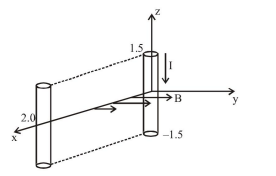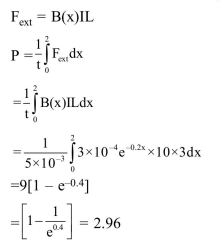Deepak Scored 45->99%ile with Bounce Back Crack Course. You can do it too!

# A conductor lies along the

Question:

A conductor lies along the $\mathrm{z}$-axis at $-1.5 \leq \mathrm{z}<1.5 \mathrm{~m}$ and carries a fixed current of $10.0 \mathrm{~A}$ in $-\hat{a}_{z}$ direction (see figure). For a field $\overrightarrow{\mathrm{B}}=3.0 \times 10^{-4} \mathrm{e}^{-0.2 \mathrm{a}} \hat{\mathrm{a}}_{\mathrm{y}} \mathrm{T}$, find the power required to move the conductor at constant speed to $\mathrm{x}=2.0 \mathrm{~m}, \mathrm{y}=0 \mathrm{~m}$ in $5 \times 10^{-3} \mathrm{~s}$. Assume parallel motion along the $\mathrm{x}$-axis.1. $14.85 \mathrm{~W}$

2. $29.7 \mathrm{~W}$

3. $1.57 \mathrm{~W}$

4. $2.97 \mathrm{~W}$

Correct Option: , 4

Solution: